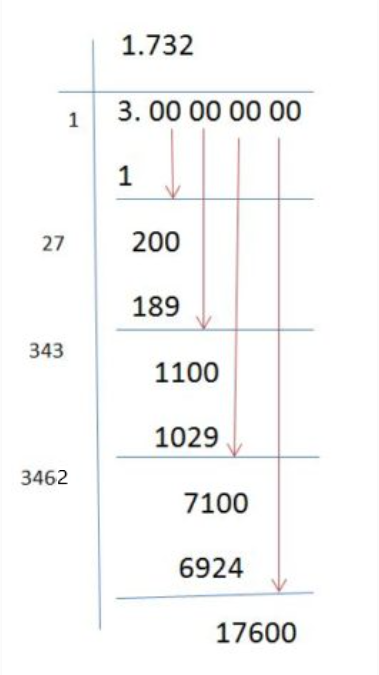# Square Root Of 3

The square root of 3  is represented using the square root or the radical symbol “√”, and it is written as √3. The value of √3 is approximately equal to 1.732. This value is widely used in mathematics. Since root 3 is an irrational number, which cannot be represented in the form of a fraction. It means that it has an infinite number of decimals. Here, we are going to have a look at the value of root and the long division method to find the value of root 3.

## Square Root of 3 Value

The square root of 3 is represented as √3 or 31/2. The numerical value of root 3 is given as follows:

√3 = 1.732050807568877293527446341505872366…..

To make the calculation easier, the value of root 3 is truncated as 1.732.

 Also,  find the values for:

## How to Find the Square Root of 3?

Generally, before finding the value for the square root of a number, it is necessary to check whether the given number is a perfect square or not. Because we can easily find the square root values for perfect squares. But, finding the square root value for non-perfect squares, is quite difficult. So, we use the long division method to find the value of the square root of 3.

## Square Root of 3 by Long Division Method

Here, we use the long division method to obtain the value of the square root of 3.Thus, the value of the square root of 3 is 1.732.

## Video Lessons on Square Roots

### Visualising square roots### Finding Square rootsThese are just the basics. There are even more complex problems to wrap your mind around. You could learn how to solve these problems and more, easily through BYJU’S – The Learning App. It explains the process in detail using illustrations and interesting examples. It also provides tips and tricks that make you think smarter.

Test your Knowledge on Square Root Of 3